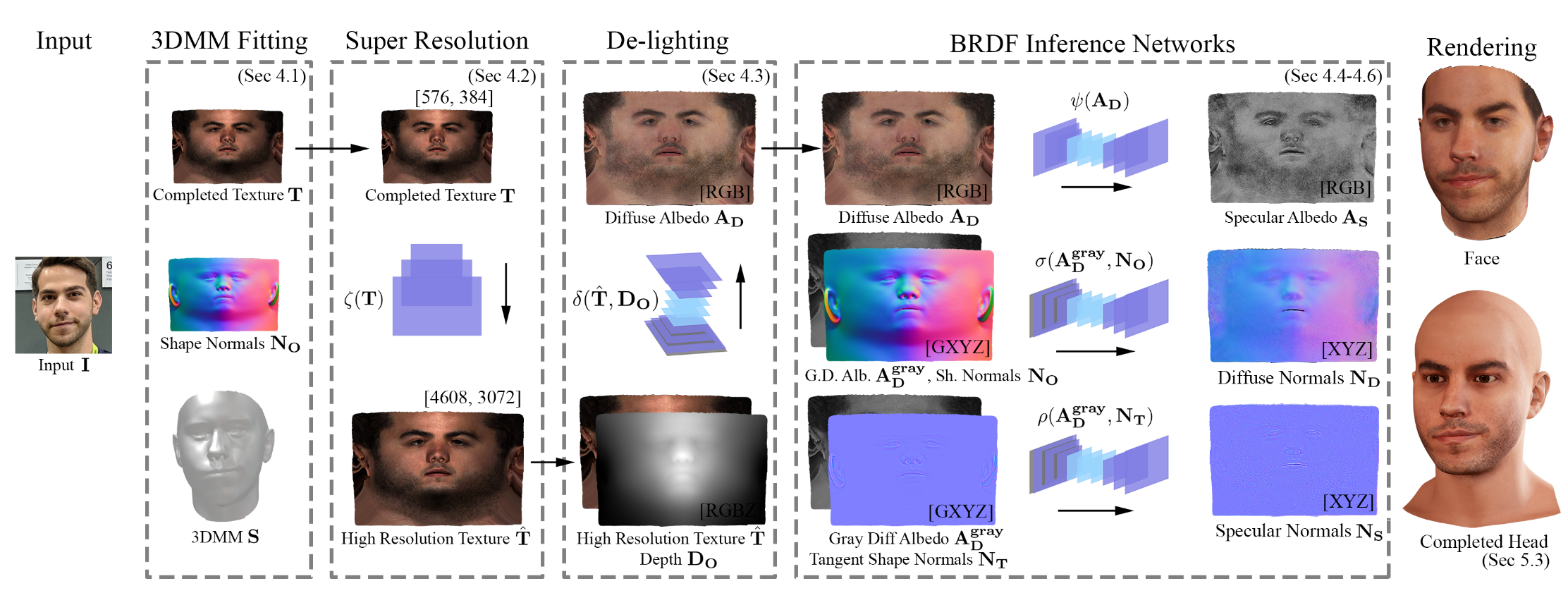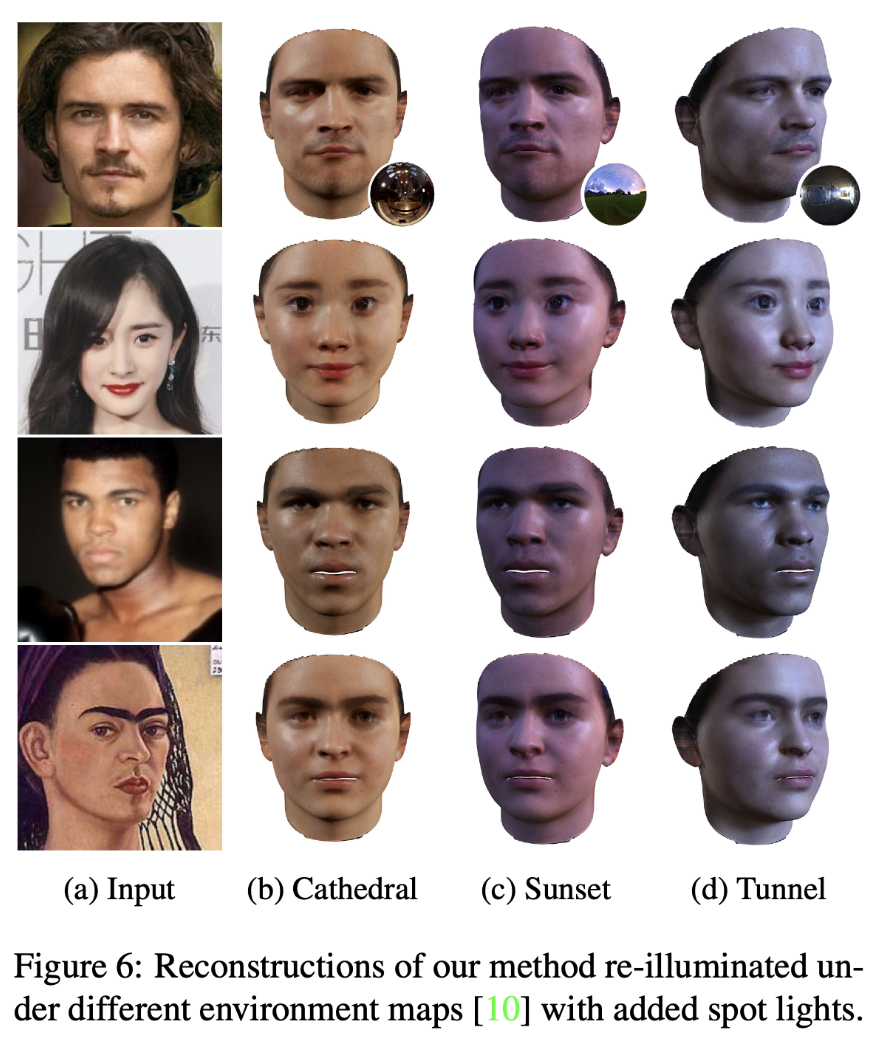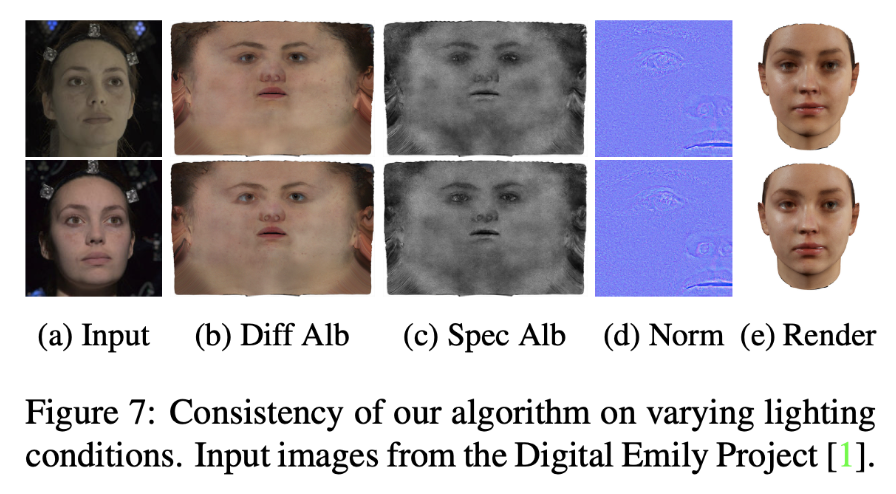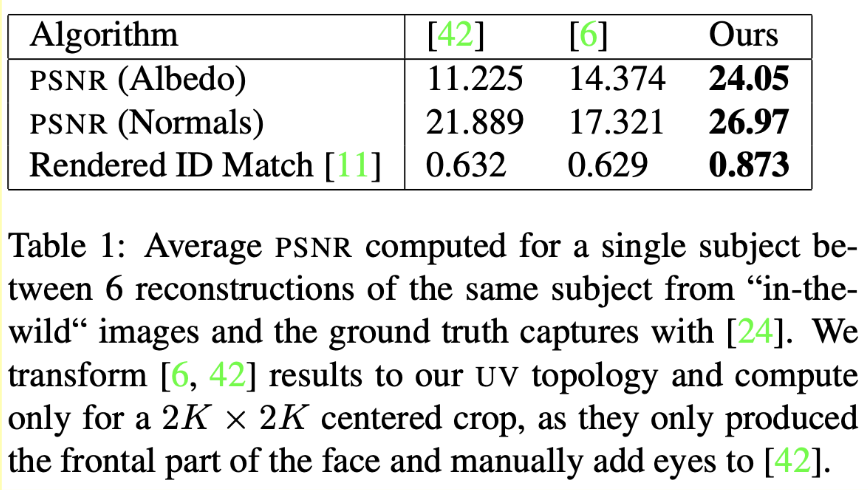# Avatarme Realistically renderable 3d facial reconstruction in-The-wild

This is reading note for Avatarme: Realistically renderable 3d facial reconstruction ‘in-The-wild’, which was published in CVPR 2020 and code is available in github. Avatarme aims to reconstruct photorealistic 3D faces from a single “in-the-wild” image with an increasing level of detail. It could generate 4K by 6K-resolution 3D faces from a single low-resolution image that, for the first time, bridges the uncanny valley.The image above shows the overview of the algorithm. To achieve photorealistic rendering of the human skin, we separately model the diffuse and specular albedo and normals of the desired geometry.

A 3DMM is fitted to an “in-the-wild” input image and a completed UV texture is synthesized, while optimizing for the identity match between the rendering and the input. The texture is up-sampled 8 times, to synthesize plausible high-frequency details. We then use an image translation network to de-light the texture and obtain the diffuse albedo with high-frequency details. Then, separate networks infer the specular albedo, diffuse normals and specular normals (in tangent space) from the diffuse albedo and the 3DMM shape normals. The networks are trained on $$512\times512$$ patches and inferences are ran on $$1536\times1536$$ patches with a sliding window. Finally, we transfer the facial shape and consistently inferred reflectance to a head model. Both face and head can be rendered realistically in any environment.

# Data

Avatarme captured high resolution pore-level reflectance maps of faces using a polarized LED sphere with 168 lights (partitioned into two polarization banks) and 9 DSLR cameras. It captured 200 individuals of different ages and characteristics under 7 different expressions.

# Algorithm Details

## Initial Geomtry and Texture via GANFIT

The initial geometry S and texture T is computed from image I via GANFIT as:

$T,S=\mathcal{G}(I)\mbox{: }T\in\mathbb{R}^{576\times384\times3},S\in\mathbb{R}^{n\times3}$

GANFIT can be described as an extension of the original 3DMM fitting strategy but with the following differences:

• instead of a PCA texture model, it uses a Generative Adversarial Network (GAN) trained on large-scale high-resolution UV-maps, and
• in order to preserve the identity in the reconstructed texture and shape, it uses features from a state-of-the-art face recognition network.

However, the reconstructed texture and shape is not render-ready due to

• the texture containing baked illumination, and
• not being able to reconstruct highfrequency normals or specular reflectance.

## Super Resolution on Texture via RCAN

The initial texture’s resolution to low for photorealistic rendering of face. To this end, Avatarme employed a state-of-the-art super-resolution network, RCAN, to increase the resolution of the UV maps from $$T\in\mathbb{R}^{576\times384\times3}$$ to $$\hat{T}\in\mathbb{R}^{4608\times3072\times3}$$, which is then retopologized and up-sampled to $$\mathbb{R}^{6144\times4096\times3}$$. Specifically, we train a super-resolution network for $$\hat{T}=\zeta(T)$$.

## Diffuse Albedo Extraction by De-lighting

Avatarme formulated de-lighting as a domain adaptation problem and train an image-to-image translation network:

• it is found find that the occlusion of illumination on the skin surface is geometry-dependent and thus the resulting albedo improves in quality when feeding the network with both the texture and geometry of the 3DMM.
• it splitted the original high resolution data into overlapping patches of $$512\times512$$ pixels in order to augment the number of data samples and avoid overfitting

This could be written as $$A_D=\delta(\hat{T},D_O)$$, where $$D_O$$ is th depth map computed from mesh S.

## Specular Albedo Extraction

A subject can be realistically rendered using only the intensity of the specular reflection $$A_S$$, which is consistent on a face due to the skins refractive index. The spatial variation is correlated to facial skin structures such as skin pores, wrinkles or hair, which act as reflection occlusions reducing the specular intensity.

Avatarme inferred the specular albedo $$A_S$$ by a similar patch-based image-to-image translation network from the diffuse albedo: $$A_S=\psi(A_D)$$.

## Specular Normals Extraction

The specular normals exhibit sharp surface details, such as fine wrinkles and skin pores, and are challenging to estimate, as the appearance of some highfrequency details is dependent on the lighting conditions and viewpoint of the texture. This is again treated as an image to image translation problem: $$N_S=\rho(A_D^{gray},N_T)$$, where $$N_S$$ is the specular normal and $$N_T$$ is the shape normals in tangent space computed from the mesh.

## Diffuse Normals Extraction

Similarly to the previous section, Avatarme trained a network to map shape normal in object space $$N_O$$ and diffusion albedo to diffuse normals: $$N_D=\sigma(A_D^{gray},N_O)$$.

# Experiment ResultsFor quantitative evaluation, PSNR is used, which is less ideal.Written on April 28, 2021